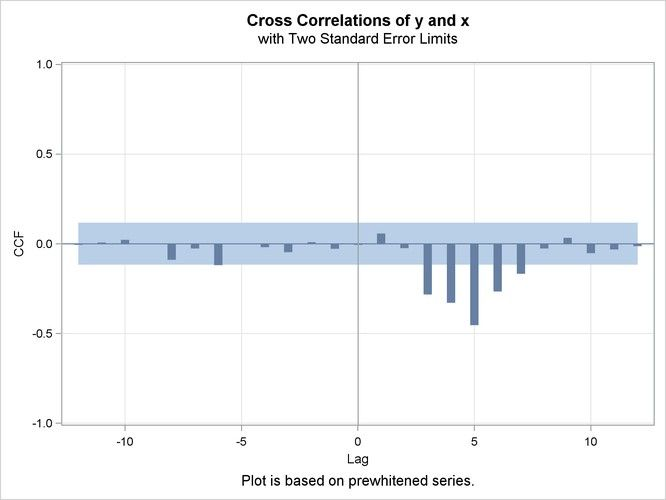## ARIMA-transfer function

As mentioned in SAS examples7.3 Model for Series J Data from Box and Jenkins, there is a simple transfer function fitted with numerator(1,2), denominator (1) and lag (3). Could anyone kindly tell me how to choose these parameters to fit transfer function model according to the Cross-correlation estimation which showed x and y had several lags (3,4,5,6,7)?1 ACCEPTED SOLUTION

Accepted Solutions

## Re: ARIMA-transfer function

Begin your reference from lag 0.

There are three intervals at lags 0, 1, and 2 before the first significant spike  ---> shift=3

A two spike build up at lags 4 and 5 following the first significant spike ---> numerator=1,2

After lag 5, there is decaying significance ---> denominator=1

If there was no decay (an immediate drop to non-significance after lag 5) ---> denominator=0

Your estimate statement in PROC ARIMA should look something like:

estimate input=(3\$(1 2)/(1)X) method=ml; run;

Once fit, evaluate the residuals for appropriate AR and MA orders and re-estimate if necessary to obtain WN residuals.

4 REPLIES 4

## Re: ARIMA-transfer function

Begin your reference from lag 0.

There are three intervals at lags 0, 1, and 2 before the first significant spike  ---> shift=3

A two spike build up at lags 4 and 5 following the first significant spike ---> numerator=1,2

After lag 5, there is decaying significance ---> denominator=1

If there was no decay (an immediate drop to non-significance after lag 5) ---> denominator=0

Your estimate statement in PROC ARIMA should look something like:

estimate input=(3\$(1 2)/(1)X) method=ml; run;

Once fit, evaluate the residuals for appropriate AR and MA orders and re-estimate if necessary to obtain WN residuals.

## Re: ARIMA-transfer function

Thanks very much for your excellent and clear reply! I have figured out this problem! Could I raise a new question that how to calculate the R-square of  a ARIMA model that we established in SAS? SAS is not the same as SPSS that can give the R-square directly. Thanks again!

## Re: ARIMA-transfer function

No problem, very glad to help.

You asked how to calculate the r-square of an ARIMA model. My first thought is why do you want to calculate r-square?

If you want a goodness-of-fit statistic to evaluate how well your model fits the data, I'd recommend choosing either AIC or SBC instead of r-square.

Unlike r-square that will never decrease as the number of model parameters increases, both AIC and SBC try to ensure the model is parsimonious. AIC and SBC are standard PROC ARIMA output when build a model.

That said, the link below walks through calculating r-square from output in PROC ARIMA.

http://support.sas.com/kb/37/955.html

## Re: ARIMA-transfer function

Thanks again for your answer. May I ask another question about the multivariate trasnfer function model? How do I choose the most parsimonious model when there are many input variables? The value of AIC or SBC change unstably when I introduce or remove some varibales? Is there any way to model the best transfer function model automatically? Thanks very much for your reply! Looking forward to hearing from you!
Discussion stats
• 4 replies
• 1483 views
• 0 likes
• 2 in conversation